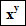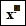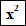Succeed with maths – Part 1

Start this free course now. Just create an account and sign in. Enrol and complete the course for a free statement of participation or digital badge if available.

Free course

5.1 Calculator exploration: exponents

Suppose you want to calculate 7 × 7 × 7 × 7. Do this on your calculator now; you should get 2401. This is fine, but as you have just seen, this calculation can be written more concisely as 74 and there is a quicker way of calculating it, too.

To work out an exponent on a calculator, you use the button(x raised to the power y).

On some calculators this will be shown asFind the correct button on your calculator now.

On most calculators you will need to enter 7, then press the exponent key followed by the 4.

Try this now for 74 and make sure you get 2401.

Table 1

 Mathematical operation Calculator button ExponentMost calculators will also have separate buttons for finding the square of a number and in some cases the cube. This will look likeand is just a short cut to finding these powers; you need to press one less button!

So far you’ve been dealing with each operator separately in a calculation but there will be occasions where you will use them in combination. In these cases you will have to know the rule that is used to ensure that everybody gets the same answer to the same calculation. This will be the subject of the next section.